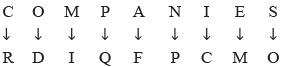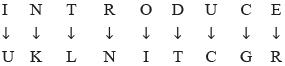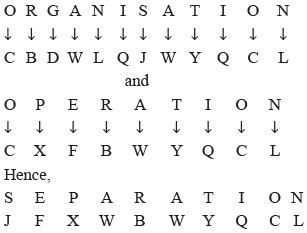Courses

# Olympiad Test: Coding Decoding -2

## 10 Questions MCQ Test Science Olympiad Class 7 | Olympiad Test: Coding Decoding -2

Description
This mock test of Olympiad Test: Coding Decoding -2 for Class 7 helps you for every Class 7 entrance exam. This contains 10 Multiple Choice Questions for Class 7 Olympiad Test: Coding Decoding -2 (mcq) to study with solutions a complete question bank. The solved questions answers in this Olympiad Test: Coding Decoding -2 quiz give you a good mix of easy questions and tough questions. Class 7 students definitely take this Olympiad Test: Coding Decoding -2 exercise for a better result in the exam. You can find other Olympiad Test: Coding Decoding -2 extra questions, long questions & short questions for Class 7 on EduRev as well by searching above.
QUESTION: 1

### In a certain code, O is written as D, A as F, M as I, S as O, N as P, E as M, I as C, P as Q and C as R, then how will COMPANIES be written in that code?

Solution:

Substituting the letters of the given word with their respective codes.QUESTION: 2

### If MINERAL is written as QRSTUVW and SOUND is written as ABCSD, then how will READER be written in the same code?

Solution:

Letter M I N E R A L S O U D
Code Q R S T U V W A B C D
The code for READER is UTVDTU.

QUESTION: 3

### If TEACHER is coded as LMKJNMP, then how will HEART be coded?

Solution:

Letter T E A C H R
Code L M K J N P
The code for HEART is NMKPL.

QUESTION: 4

If R is denoted by N, D is denoted by T, I by U, O by I, E by R, T by L, U by C, N by K and C by G, then how will the word INTRODUCE be written?

Solution:

Substituting the letters of the given word with their respective codes,QUESTION: 5

If the word PORTER can be coded as MBNZQN. How can REPORT be written?

Solution:

Letter P O R T E
Code M B N Z Q
The code for REPORT is NQMBNZ.

QUESTION: 6

If the word RASCALS can be coded as BNMZMTN. How can SALSA be written?

Solution:

Letter R A S C L
Code B N M Z T
The code for SALSA is MNTMN.

QUESTION: 7

If in a code language, ORGANISATION is written as CBDWLQJWYQCL and OPERATION is written as CXFBWYQCL, then how is SEPARATION coded?​

Solution:QUESTION: 8

In a code language, FACTORIAL is written as DODNGLCFH and DANCE is written as YCJMZ, how can EDUCATION be written in that code?

Solution:

Letter F A C T O R I L D N E
Code D O D N G L C H Y J Z
The code for EDUCATION is ZJDONCGJ.

QUESTION: 9

In a coding system,  SHEEP is written as GAXXR and BLEAT as HPXTN. How can SLATE be written in this coding system?

Solution:

Letter SH E P B L A T
Code GA X R H P T N
The code for SLATE is GPTNX.

QUESTION: 10

In a code language, STARK is written as LBFMG and MOBILE is written as TNRSPJ. How is BLAME written in that code?

Solution:

Letter S T A R K M O B I L E
Code L B F M G T N R S P J
The code for BLAME is RPFTJ.